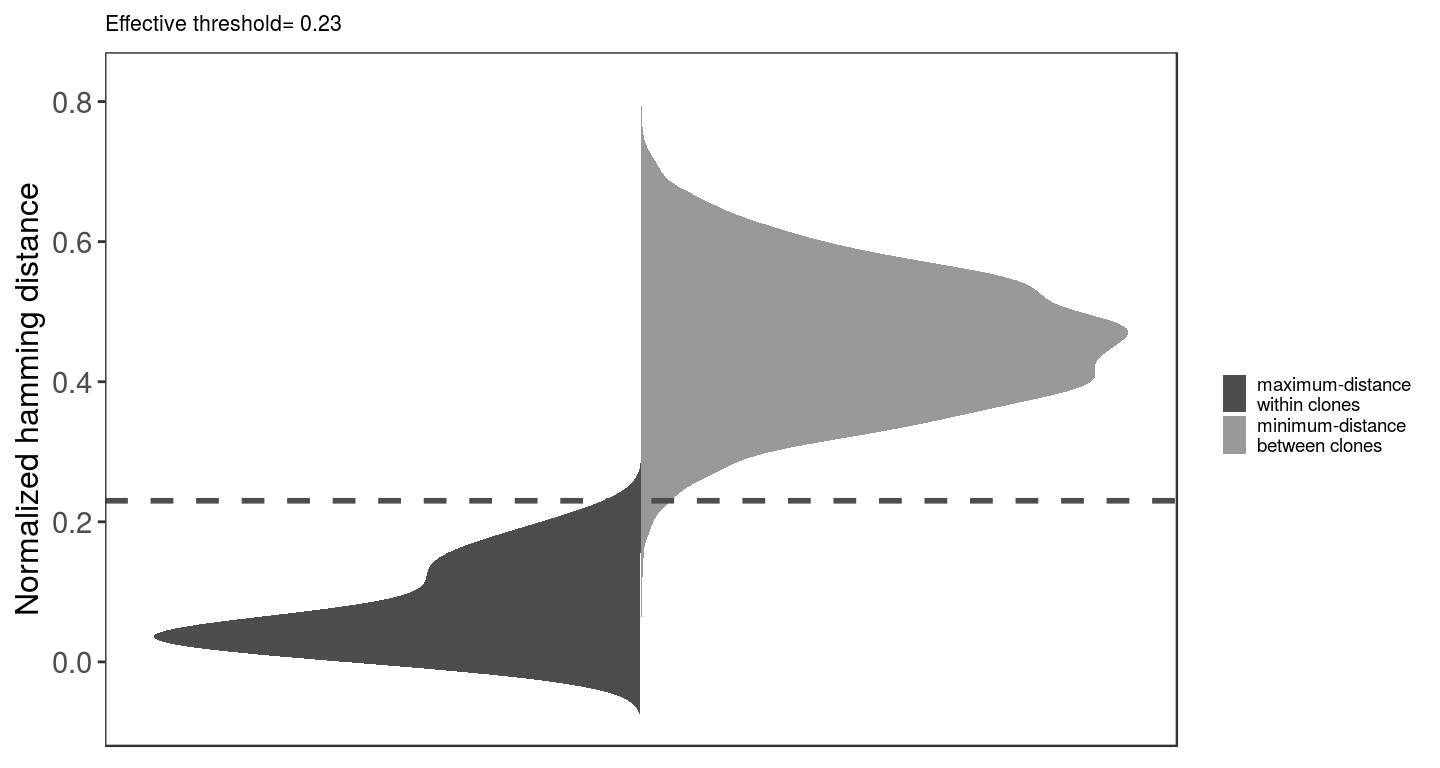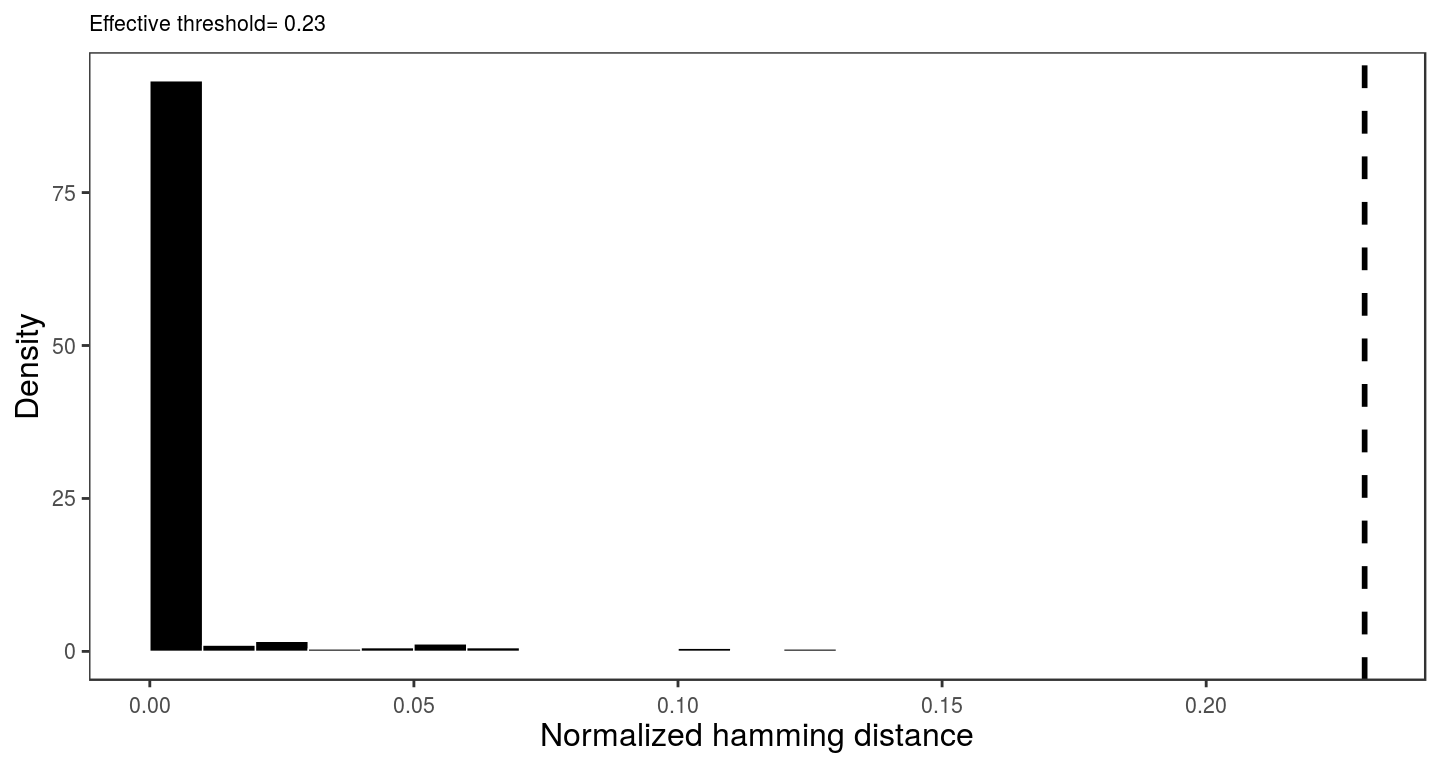## Description

scoper package provides a computational framework for unsupervised identification B cell clones from adaptive immune receptor repertoire sequencing (AIRR-Seq) datasets. This method is based on spectral clustering of the junction sequences of B cell receptors (BCRs, Immunoglobulins) that share the same V gene, J gene and junction length.

## Method

The spectral clustering-based method proceeds in five steps, as follows:

1. Compute the similarity matrix: Given a set of BCR sequences $$\{x_1, x_2, \cdots, x_n\}$$ we generate a symmetric matrix with entries $$s_{ij}$$ defined by the Hamming distance between the junction regions of sequences $$x_i$$ and $$x_j$$.

2. Compute the kernel matrix: Given the $$(n,n)$$ similarity matrix, we generate a fully connected graph such that its elements represent the local neighborhood relationship of each sequence to all other sequences (i.e., edges between sequences in local neighborhoods are connected with relatively high positive weights, while edges between far away sequences have smaller positive weights). This is implemented using a Gaussian kernel matrix with elements $$k_{ij} = \exp(-s^2_{ij}/2\sigma_i \sigma_j)$$, where the parameters $$\sigma_i$$ and $$\sigma_j$$ (standard deviation) control the width of the neighborhoods corresponding to the sequences $$x_i$$ and $$x_j$$, respectively. The standard deviation is computed such that the width of neighborhood varies in different parts of the graph capturing a dynamic threshold among only those junction segments which have shown higher similarity than the other sequences. To calculate the scale parameter $$\sigma_i$$, the rank-ordered set of distances corresponding to the $$i^\textrm{th}$$ row of the similarity matrix $$s$$ is examined to find the first largest gap in distance values. This gap is flagged as the neighborhood width. Finally, we compute the scale parameter $$\sigma_i$$ associated with $$i^\textrm{th}$$ sequence as the standard deviation of distances within this neighborhood.

3. Compute the Laplacian matrix: Given the $$(n,n)$$ kernel matrix we generate graph Laplacian defined as $$L=D-K$$, where $$D$$ is a diagonal matrix defined as $$D_{ii}=\sum_j A_{ij}$$. Subsequently, we calculate the eigenvectors and eigenvalues of this matrix.

4. Determine the number of clusters: Given the set of eigenvalues $$\{0=\lambda_1\le \lambda_2\le \cdots\le \lambda_n\}$$ we infer the number of clusters, k, such that all eigenvalues $$\lambda_1, \cdots, \lambda_k$$ are very small ($$\simeq\!0$$), but $$\lambda_{k+1}$$ is relatively large. Therefore, the rank-ordered eigenvalues are examined to find the first largest gap where $$\lambda_{k+1}>0$$, while the eigenvalue $$\simeq0$$ has multiplicity up to $$k^{th}$$ eigenvalue. Then, the value $$k$$ is used as the number of clusters.

5. Clonal inference: Given the number of clusters $$k$$, we perform $$k$$-means Euclidean distance-based clustering over the $$k$$ eigenvectors associated with the smallest $$k$$ eigenvalues to find the appropriate clones.

## Requirements

Clonal family inference using spectral clustering-based technique requires the following fields (columns) to be present in the Change-O database:

• V_CALL
• J_CALL
• JUNCTION
# Load scoper
library("scoper")

## Clonal partitioning

The function for the clonal family inference takes a few parameters:

defineClonesScoper(db, junction = "JUNCTION", v_call = "V_CALL", j_call = "J_CALL",
first = FALSE, cdr3 = FALSE, mod3 = FALSE, iter_max = 1000,
nstart = 25, nproc = 1, progress = FALSE, out_name = NULL,
out_dir = ".")

The data set needs to be passed to the argument db, which at the end would be returned as a modified db data.frame with clone identifiers in the CLONE column. Name of the columns containing nucleotide sequences (junction region), the V-segment allele calls, and the J-segment allele calls needs to be assigned to the arguments junction, v_call, and j_call, respectively. If a genotype has been inferred using the methods in the tigger package, and a V_CALL_GENOTYPED field has been added to the database, then this column may be used instead of the default V_CALL column by specifying the v_call argument. This will allows the more accurate V call from tigger to be used for grouping of the sequences. Furthermore, for more leniency toward ambiguous V(D)J segment calls, the parameter first can be set to FALSE. To remove $$3$$ nucleotides from both ends of the junction region (i.e., converting IMGT junction to Complementarity-Determining Region $$3$$ region) the logical argument cdr3 needs to be set as TRUE (the default is set to be FALSE). This also leads to the removal of junction(s) with length less than $$7$$ nucleotides from the original db dataset. To remove junction(s) with number of nucleotides not modulus of $$3$$, the logical argument mod3 should be set as TRUE (the default is set to be FALSE). Arguments iter_max and nstart are required to perform the k-means clustering step of the pipeline. They will pass, respectively, the maximum allowed number of kmean clustering iterations and the number of random sets chosen for kmean clustering initialization. Finally, if the argument out_name be assigned, a *.tsv Change-O formated of cloned data.frame and a summary of cloning performance would be saved in the current directory. The out_name string is used as the prefix of the successfully processed output files. User can also asign the desire directory path to the out_dir argument. A small example Change-O database is included in the scoper package:

# Clone data using defineClonesScoper function
db <- defineClonesScoper(ExampleDb, junction = "JUNCTION", v_call = "V_CALL",
j_call = "J_CALL", first = TRUE)
## CLONES=  1058
## RECORDS=  2000
## PASS=  2000
## FAIL=  0

## Clonal analysis

To perform a series of analysis to assess the cloning performance, analyzeClones function has been developed:

analyzeClones(db, junction = "JUNCTION", v_call = "V_CALL", j_call = "J_CALL",
clone = "CLONE", first = FALSE, cdr3 = FALSE, nproc = 1,
progress = FALSE), warning=FALSE, message=FALSE

The analyzeClones function invokes the cloned db data.frame and provides summary statistics and visualization of the clonal clustering results. The arguments junction, v_call, j_call, first, and cdr3 should match the parameters which were passed to the defineClonesScoper function and the name of the clone identifier column is invoked by the argument clone. After analyzing the analyzeClones function returns an R object including infromation to interpret clonal assignment performance:

1. threshold: effective cut-off separating the inter (within) and intra (between) clonal distances.
2. inter_intra: data.frame containing all inter and intra clonal distances.
3. plot_inter_intra: density plot of inter versus intra clonal distances. The threshold is shown with a horizental dashed-line.
4. neighborhoods: numeric vector containing scale parameters used in spectral clustering process.
5. plot_neighborhoods: histogram of neighborhoods. The effective threshold is shown with a vertical dashed-line.

A small example Change-O database is included in the scoper package:

# Clonal assignment analysis
results <- analyzeClones(ClonedExampleDb, junction = "JUNCTION", v_call = "V_CALL",
j_call = "J_CALL", clone = "CLONE", first = TRUE)
# print threshold (a numeric)
results@threshold
##  0.23
# get inter and intra conal distances (a data.frame)
df <- results@inter_intra[]
# density plot of inter versus intra clonal distances  (a ggplot).
results@plot_inter_intra
## []# get the neighborhoods used in spectral clustering (a numeric vector).
ngs <- results@neighborhoods
# plot histogram of neighborhoods (a ggplot).
results@plot_neighborhoods
## []